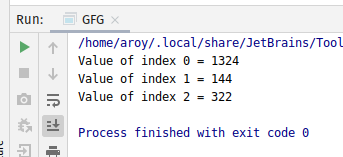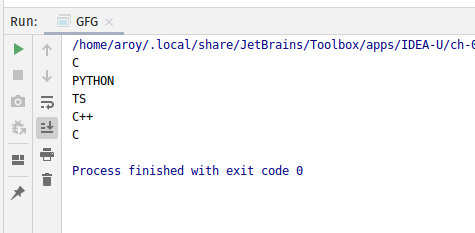# AtomicReferenceArray setOpaque() method in Java with Examples

• Last Updated : 03 Jan, 2020

The setOpaque() method of a AtomicReferenceArray class is used to set the value of the element at index i to newValue. Both index i and newValue are passed as parameters to the method. This method set the value with memory effects as specified by VarHandle.setOpaque(java.lang.Object…). In this way value is set in program order, but with no assurance of memory ordering effects with respect to other threads.

Syntax:

```public final void setOpaque(int i, E newValue)
```

Parameters: This method accepts:

• i which is an index of AtomicReferenceArray to perform the operation,
• newValue which is the new value to set.

Return value: This method returns nothing.

Below programs illustrate the setOpaque() method:
Program 1:

 `// Java program to demonstrate``// setOpaque() method`` ` `import` `java.util.concurrent.atomic.*;`` ` `public` `class` `GFG {`` ` `    ``public` `static` `void` `main(String[] args)``    ``{`` ` `        ``// create an atomic reference object.``        ``AtomicReferenceArray ref``            ``= ``new` `AtomicReferenceArray(``5``);`` ` `        ``// set some value and print``        ``ref.setOpaque(``0``, ``1324``);``        ``ref.setOpaque(``1``, ``144``);``        ``ref.setOpaque(``2``, ``322``);`` ` `        ``System.out.println(``"Value of index 0 = "``                           ``+ ref.get(``0``));``        ``System.out.println(``"Value of index 1 = "``                           ``+ ref.get(``1``));``        ``System.out.println(``"Value of index 2 = "``                           ``+ ref.get(``2``));``    ``}``}`

Output:Program 2:

 `// Java program to demonstrate``// setOpaque() method`` ` `import` `java.util.concurrent.atomic.*;`` ` `public` `class` `GFG {`` ` `    ``public` `static` `void` `main(String[] args)``    ``{`` ` `        ``// create an atomic reference object.c``        ``AtomicReferenceArray ref``            ``= ``new` `AtomicReferenceArray(``5``);`` ` `        ``// set some value``        ``ref.setOpaque(``0``, ``"C"``);``        ``ref.setOpaque(``1``, ``"PYTHON"``);``        ``ref.setOpaque(``2``, ``"TS"``);``        ``ref.setOpaque(``3``, ``"C++"``);``        ``ref.setOpaque(``4``, ``"C"``);`` ` `        ``// print``        ``for` `(``int` `i = ``0``; i < ``5``; i++) {``            ``System.out.println(ref.get(i));``        ``}``    ``}``}`

Output:My Personal Notes arrow_drop_up